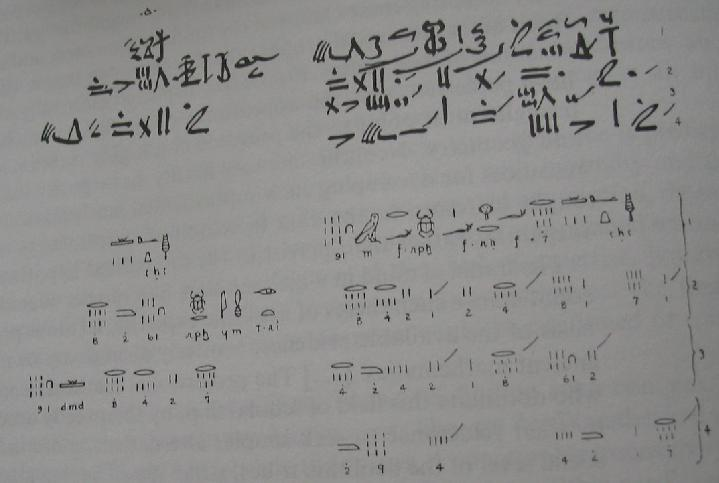# Rhind Papyrus

The Rhind Mathematical Papyrus [Midonick, pp. 706-732, Fauvel, pp. 14-16] is the largest among the existing Egyptian papyri. It's named after the British archeologist A. Henry Rhind who purchased it in 1858. It was acquired by the British Museum in 1864 from Rhind's estate and made available in facsimile form to scholars of mathematics and Egyptology. Originally it came in two parts of a single scroll. The missing (middle) part was discovered in 1922 among a private papyri collection in New York. The Rhind Papyrus which is dated circa 1650 B.C. contains some problems that are found in an ealier Moscow Papyrus dating from 1850 B.C.

Much of the Rhind Papyrus deals with proportional reasoning and multiplication by doubling. I'll give a couple of examples based on [Midonick].

#### Problem 4

Divide 7 loaves among 10 men.

#### Proof

 Do it thus: 1 2/3 1/30 \2 1 1/3 1/15 4 2 2/3 1/10 1/30 \8 5 1/2 1/10

Total of 7 loaves, which is correct.

#### Commentary

The expression "2/3 1/30" should be understood as the sum of terms involved. In the modern notations: 2/3 + 1/30 = 21/30 = 7/10, which is of course the correct result. There is no explanation how the author has obtained it. But we do know that the Egyptian have been especially adept at representing numbers as sums of distinct fractions having unit numerators (2/3 being an exception.)

That expression, representing 1 part of the sought quantity is then doubled, and the result is doubled again to obtain the representation of 4 units and then that of 8 units. Since 10 = 2 + 8, the two lines corresponding to 2 and 8 units are marked with "\" and then added:

 10 = 2 + 8 = 1 + 1/3 + 1/15 + 5 + 1/2 + 1/10 = 6 + 10/30 + 2/30 + 15/30 + 3/30 = 6 + (10 + 2 + 15 + 3)/30 = 6 + 1 = 7.

The foregoing example must be taken with a grain of salt. Written in the modern notations, it conveys the spirit of the procedure. In particular, it is noteworthy that multiplication by 10 has been carried out by repeated doubling and addition, which links it to the Russian Peasant Multiplication algorithm.

A reproduction below gives a better notion of the appearance of the manuscript. (The top part is a real portion of the scroll. The bottom part is the interpreter's rendering of the text above it.)

#### Problem 24A quantity and its 1/7 added together become 19. What is the quantity?

Assume 8.

 \1 7 \1/7 1 Total 8

As many times as 8 must be multiplied to give 19, so many times 7 must be multiplied to give the required number.

 1 8 \2 16 1/2 4 \1/4 2 \1/8 1 Total 2 1/4 1/8 \1 2 1/4 1/8 \2 4 1/2 1/4 \4 9 1/2
 Do it thus: 1 16 1/2 1/8 1/7 2 1/4 1/8 Total 19.

You may want to compare this to a modern day solution.

### References

1. J. Fauvel, J. Gray (eds), The History of Mathematics. A Reader, The Open University, 1987
2. H. O. Midonick (ed), The Treasury of Mathematics, Philosophical Library, 1965